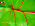## Thursday, September 25, 2008

### Simple Graphics Calculator Using the Visualization API and the Scatterchart

We recently came across a great use of the Visualization Platform. In fact, this is something that we never thought the platform would be used for.

Steve Aitken, a developer contributing to the Visualization Developer Group, created a simple graphics calculator for Javascript-supported math functions that plots functions using the Google Visualization Scatter Chart. Here is a screenshot of a simple calculation of -sin(2x):Steve has been kind enough to share the code with us (even though it was originally written for his girlfriend). A slightly modified version is pasted below:
``<html>  <head>    <script type="text/javascript" src="http://www.google.com/jsapi"></script>    <script type="text/javascript">      google.load("visualization", "1", {packages:["scatterchart"]});      function drawChart(equation,xmin,xmax, numPoints, pointSize) {        var data = new google.visualization.DataTable();        data.addColumn('number', 'x');        data.addColumn('number', 'y');        data.addRows(numPoints);        var step = (xmax-xmin) / (numPoints-1);        for(var i = 0; i < numPoints; i++)        {          var x = xmin + step * i;          data.setValue(i,0,x);          with(Math) {            var y = eval(equation);          }          data.setValue(i,1,y);        }        document.getElementById("chart_div").innerHTML = "";        var chart = new google.visualization.ScatterChart(            document.getElementById('chart_div'));        chart.draw(data, {width: 600, height: 400, titleX: 'X',            titleY: 'Y', legend: 'none', pointSize: pointSize});      }    </script>  </head>  <body>      equation: &nbsp; &nbsp; &nbsp; &nbsp; &nbsp; &nbsp; &nbsp;      <input id="txteq" type="text" value="-sin(2*x)" />      <br />      minimum value(x): &nbsp;<input id="txtmin" type="text" value="-3.14" />      <br />      maximum value(x): &nbsp;<input id="txtmax" type="text" value="3.14"/>      <br />      Precision (number of points): &nbsp;<input id="precision" type="text" value="1000"/>      <br />      Point size: &nbsp; <input id="pointSize" type="text" value="2"/>      <br />      <input id="Button1" type="button" value="Draw Graph"          onclick="javascript:drawChart(              document.getElementById('txteq').value,              parseFloat(document.getElementById('txtmin').value, 10),              parseFloat(document.getElementById('txtmax').value, 10),              parseInt(document.getElementById('precision').value, 10),              parseInt(document.getElementById('pointSize').value, 10))" />    <div id="chart_div"></div>  </body></html>``

We thank Steve for the inspiration and would love to see more creative uses of the platform from you.

The Visualization Team

#### 6 comments:

1.It's been done... http://grapher.greenmangames.vze.com

2.'with(Math) var y = eval(equation)' is a really bad way to achieve this. A (marginally) better solution would be to do something akin to:

var expressionFunction;
with(Math) expressionFunction = eval("(function(x){"+expression +"})");

then replace the later use of "with(Math)eval(expression)" with expressionFunction(x);

3.If I have the following data set:

data.addColumn('string', 'Date'); data.addColumn('number', 'Index');

data.setValue(0, 0, dates);
data.setValue(1, 1, 75.5678);

data.setValue(1, 0, dates);
data.setValue(1, 1, 75.6934);

data.setValue(2, 0, dates);
data.setValue(2, 1, 75.9932);

data.setValue(3, 0, dates);
data.setValue(3, 1, 76.0198);

data.setValue(4, 0, dates);
data.setValue(4, 1, 75.4293);

The visualization that is generated whether it is an interactive line
graph or a plain vanilla line graph is essentially a straight line.

Now if I could set the level of "granularity" on the graph so that I
could actually see the differences in the points plotted, then my
problem is solved.

Or, can I set where the default value is for the y-axis? I searched
here on the group and it appears I cannot.

Any help is greatly appreciated.

4.Math Mechanixs is an easy to use general purpose math program. It has a Math Editor worksheet for solving mathematical problems and an extendable Function Library containing over 170 predefined functions with an integrated Function Solver.
---------------------
Selleys

forum post

5.Pretty cool! Here is a live copy for anyone to play with.

@Oliver
Could you update the code with your suggestion? I tried to implement that change, but wasn't sure what was supposed to go where.

6.@mike chelen: I've posted a modified version of your live demo at http://nerget.com/google_graph_vis_doofer.html

My very ad hoc testing shows the function version to be 3-5x faster than calling eval over and over. There are a number of reasons for this. The most obvious is that you don't have to reparse and compile the eval argument over and over again. But other advantages cover things like variable access performance.

eg. function(x) { return x*x; } can statically bind both references to x, whereas using eval prevents that by introducing a dynamic scope.

Alas even the use of with(Math) harms performance, however by defining the function as:
with(Math) (function(x){ return ...; }) we can remove the dynamic lookup cost for anything other than actual uses of the Math object.

A more performant (albeit memory hungry) approach would be to something akin to:
var mathStr = "var sin = Math.sin, cos=Math.cos, ...."
then create the function with "window.eval(mathStr + "(function(x){ return "+equation+";})")

Which would result in a static lookup for the Math functions.